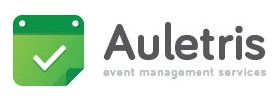## August 22 - August 30, 2020

### /* RAINBOW TEXT Script by Matt Hedgecoe (c) 2002 Featured on JavaScript Kit For this script, visit http://www.javascriptkit.com */ // ********** MAKE YOUR CHANGES HERE var text="Registration system opened for pre-registration" // YOUR TEXT var speed=100 // SPEED OF FADE // ********** LEAVE THE NEXT BIT ALONE! if (document.all||document.getElementById){ document.write('<span id="highlight">' + text + '</span>') var storetext=document.getElementById? document.getElementById("highlight") : document.all.highlight } else document.write(text) var hex=new Array("00","14","28","3C","50","64","78","8C","A0","B4","C8","DC","F0") var r=1 var g=1 var b=1 var seq=1 function changetext(){ rainbow="#"+hex[r]+hex[g]+hex[b] storetext.style.color=rainbow } function change(){ if (seq==6){ b-- if (b==0) seq=1 } if (seq==5){ r++ if (r==12) seq=6 } if (seq==4){ g-- if (g==0) seq=5 } if (seq==3){ b++ if (b==12) seq=4 } if (seq==2){ r-- if (r==0) seq=3 } if (seq==1){ g++ if (g==12) seq=2 } changetext() } function starteffect(){ if (document.all||document.getElementById) flash=setInterval("change()",speed) } starteffect() /* Count down until any date script- By JavaScript Kit (www.javascriptkit.com) Over 200+ free scripts here! */ //change the text below to reflect your own, var before="to the conference opening" var current="We are starting today" var montharray=new Array("Jan","Feb","Mar","Apr","May","Jun","Jul","Aug","Sep","Oct","Nov","Dec") function countdown(yr,m,d){ var today=new Date() var todayy=today.getYear() if (todayy < 1000) todayy+=1900 var todaym=today.getMonth() var todayd=today.getDate() var todaystring=montharray[todaym]+" "+todayd+", "+todayy var futurestring=montharray[m-1]+" "+d+", "+yr var difference=(Math.round((Date.parse(futurestring)-Date.parse(todaystring))/(24*60*60*1000))*1) if (difference==0) document.write(current) else if (difference>0) document.write(""+difference+" days "+before) } //enter the count down date using the format year/month/day countdown(2020,8,22)